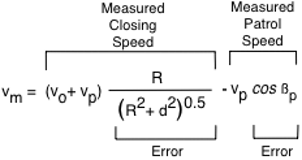Previous | Next

# Moving Radar Cosine Effect Microwave Radar

Contents -- Cosine Effect Error | Minimum Range
 Moving Mode Cosine Effect Error

The main difference between stationary and moving mode radar is moving radar also measures patrol vehicle speed by measuring the ground reflection speed. A moving radar measures traffic speed relative to the radar, closing speed for on-coming traffic. Patrol vehicle speed is subtracted from closing speed to obtain traffic speed.

Closing Speed
vc = vo + vp
Target Vehicle Speed
vo = vc - vo
vc = Closing Speed, vp = Patrol Speed, vo = target vehicle speed

Moving mode radar antennas are designed to be aimed exactly in the direction of travel, directly forward for front antennas and directly aft for rear antennas. An off angle ground echo measures patrol speed low by the product of the cosine angle resulting in a traffic speed error, Patrol Speed Shadowing by definition.

 vpm = vp cos ß

 vpm = Radar Measured Patrol Speed vp = Actual Radar Patrol Speed ß = Ground Echo Angle Error

A patrol vehicle speed that measures low will calculate target vehicle speed high by the speed the patrol vehicle is low.

The radar identifies the main beam center ground echo as the strongest echo, it usually is by several orders of magnitude. Sometimes stronger reflections come from off angle that may be at the main beam edge instead of center, or may not be in the main beam at all but in a side lobe.

Opposite Direction Traffic
The cosine effect potentially introduces 2 errors in moving mode. One error is the angle between antenna and target vehicle which measures target speed low. The other error is the angle of the ground echo, the greater the angle the lower measured patrol speed and the higher the measured target speed.

Moving Mode Speed Angle Errorsvm = measured traffic speed vo = traffic speed vp = patrol vehicle speed ß = cosine effect angle ßp = ground echo angle error

Measured Speed Errorvm = measured traffic speed vo = traffic speed vp = patrol vehicle speed R = vehicle range to radar d = radar distance from traffic lane ßp = ground echo angle error

Cosine Effect Acceleration
The cosine effect on acceleration for moving mode is the same as for stationary operation except the patrol vehicle radar and traffic are approaching, or receding, each other. The cosine effect angle is changing at the rate proportional to not just traffic speed but also the patrol vehicle speed. The acceleration equation for stationary radar applies to moving mode radar by substituting closing or opening speed for traffic speed.a = cosine effect acceleration vo = traffic speed vp = patrol vehicle speed R = vehicle range to radar d = radar distance from traffic lane

 Moving Mode Minimum Range

Moving mode radar acceleration limit is a function of radar patrol speed accuracy and minimum sample time. Typically traffic accuracy is ±2 mph and patrol accuracy is ±1 mph. The radar's traffic speed measurement accuracy for moving mode is double that of stationary mode because the radar is using 2 measurements, radar speed and closing or oening speed to derive traffic speed - 2 error sources.

 amax = ±vacc / ti
 amax = radar acceleration limit vacc = radar accuracy ti = radar sample time or integration period

Minimum range is a function of;
 Traffic Speed (Vo) Patrol Vehicle Speed (Vp) Stationary Mode Accuracy (±Vacc) Integration Period or sample time (ti) Antenna Distance from Traffic Lane Center (d)

Minimum Range
0.300 second Sample Time, ±1 mph AccuracyMinimum Range
0.250 second Sample Time, ±1 mph AccuracyMinimum Range Factors Traffic Speed Patrol Vehicle Speed Antenna Distance from Traffic Lane Radar Patrol Speed Accuracy (Typically ±1 mph) Radar Sample TIme (Typically 0.3 seconds)

Moving Mode Minimum Range EquationRm = minimum range vo = traffic speed vp = patrol vehicle speed vacc = patrol speed accuracy ti = radar sample time d = antenna distance to traffic lane

Minimum Range
Traffic closer than minimum range cannot be measured.
 vo = Traffic Speed: mph vp = Patrol Vehicle Speed: mph d = Radar to Traffic Lane: feet ti = Radar Sample Time: seconds milliseconds vacc = Stationary Mode Accuracy: ± 1 mph Minimum RangeTime to Even with Radar

Password section -- Cosine Effect Simulator